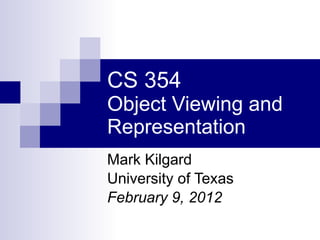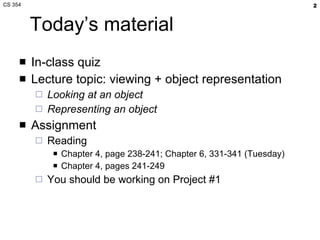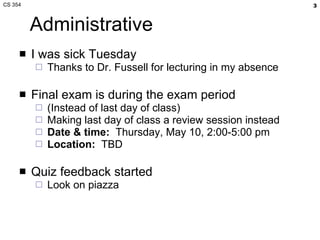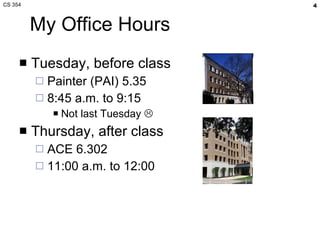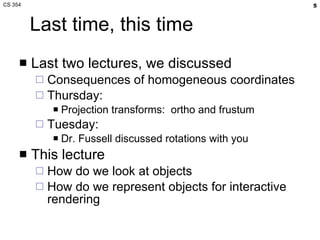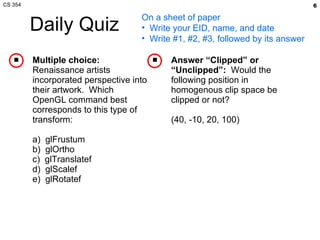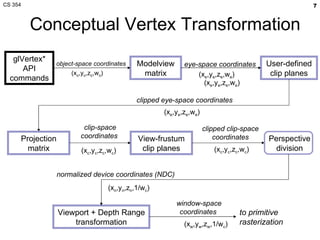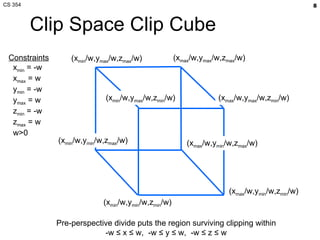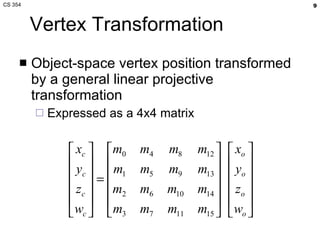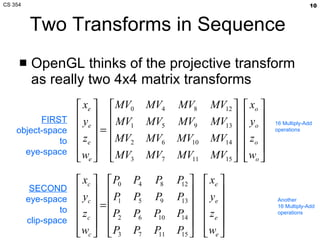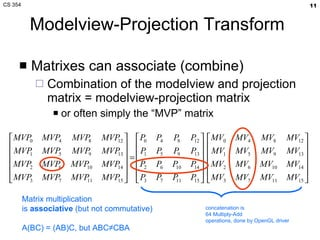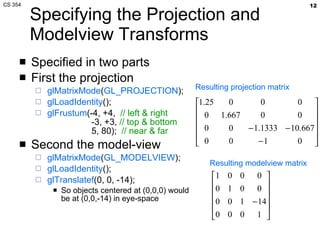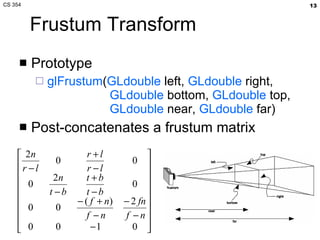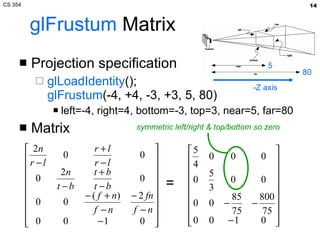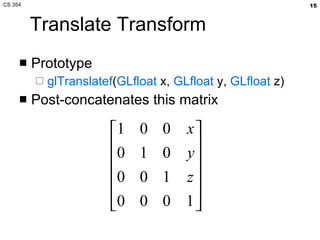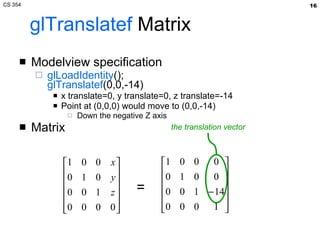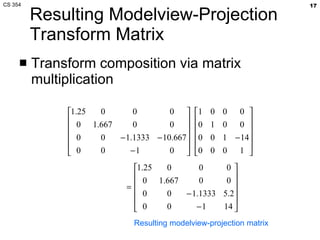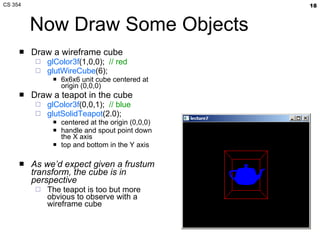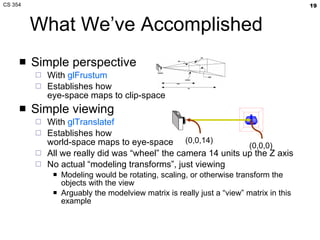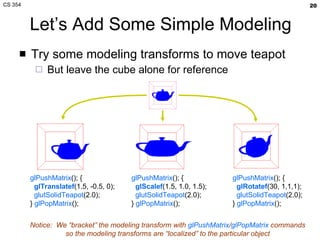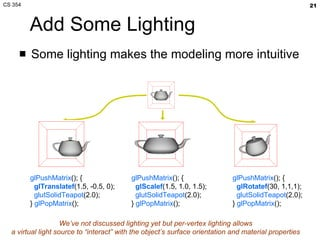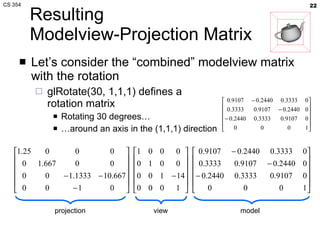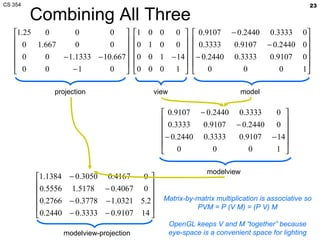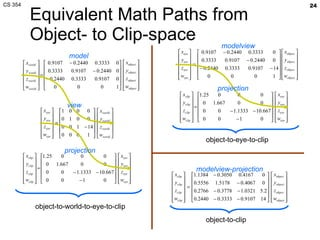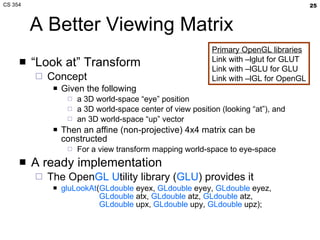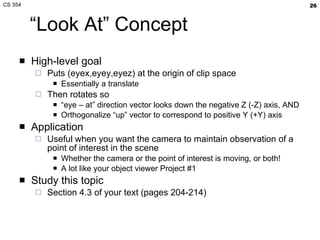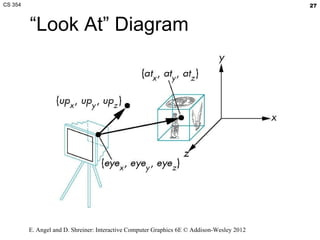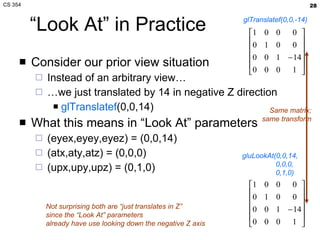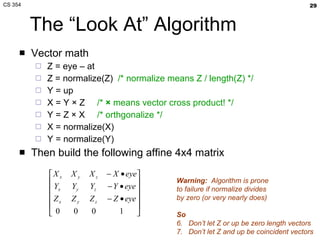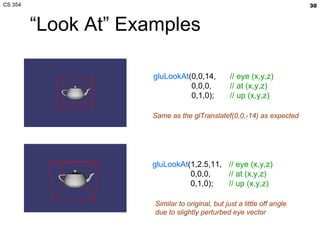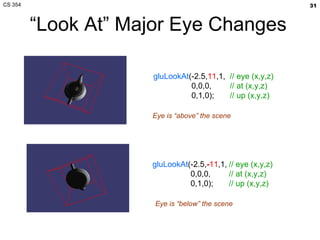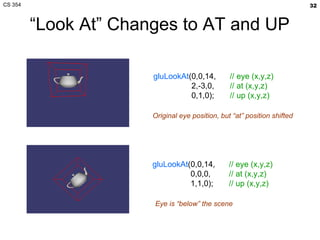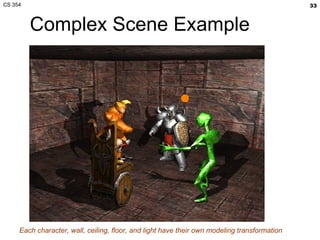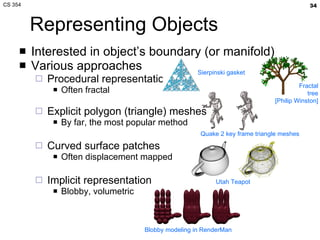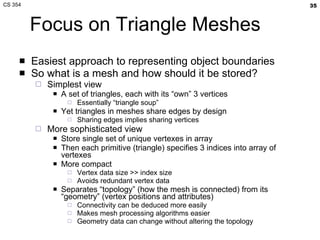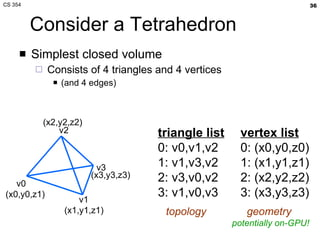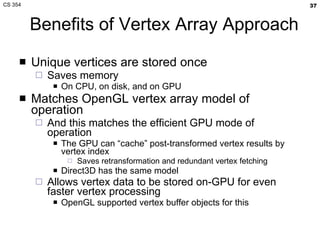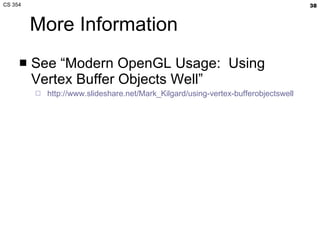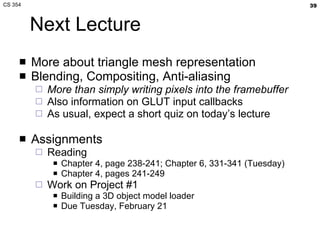1 of 39

### CS 354 Object Viewing and Representation

1. CS 354 Object Viewing and Representation Mark Kilgard University of Texas February 9, 2012
2. Conceptual Vertex Transformation glVertex* API commands Modelview matrix User-defined clip planes View-frustum clip planes to primitive rasterization object-space coordinates (x o ,y o ,z o ,w o ) eye-space coordinates (x e ,y e ,z e ,w e ) clipped eye-space coordinates clipped clip-space coordinates Perspective division Projection matrix Viewport + Depth Range transformation (x c ,y c ,z c ,w c ) window-space coordinates (x w ,y w ,z w ,1/w c ) normalized device coordinates (NDC) (x n ,y n ,z n ,1/w c ) clip-space coordinates (x c ,y c ,z c ,w c ) (x e ,y e ,z e ,w e ) (x e ,y e ,z e ,w e )
3. Clip Space Clip Cube (x min /w,y min /w,z min /w) Pre-perspective divide puts the region surviving clipping within -w ≤ x ≤ w, -w ≤ y ≤ w, -w ≤ z ≤ w (x max /w,y min /w,z min /w) (x max /w,y min /w,z max /w) (x min /w,y min /w,z max /w) (x max /w,y max /w,z max /w) (x max /w,y max /w,z min /w) (x min /w,y max /w,z min /w) (x min /w,y max /w,z max /w) Constraints x min = -w x max = w y min = -w y max = w z min = -w z max = w w>0
4. Combining All Three projection view model modelview modelview-projection Matrix-by-matrix multiplication is associative so PVM = P (V M) = (P V) M OpenGL keeps V and M “together” because eye-space is a convenient space for lighting
5. Equivalent Math Paths from Object- to Clip-space object-to-world-to-eye-to-clip object-to-eye-to-clip object-to-clip modelview projection projection modelview-projection model view
6. “Look At” Diagram E. Angel and D. Shreiner: Interactive Computer Graphics 6E © Addison-Wesley 2012
7. “Look At” Examples gluLookAt (0,0,14, 0,0,0, 0,1,0); // eye (x,y,z) // at (x,y,z) // up (x,y,z) gluLookAt (1,2.5,11, 0,0,0, 0,1,0); // eye (x,y,z) // at (x,y,z) // up (x,y,z) Same as the glTranslatef(0,0,-14) as expected Similar to original, but just a little off angle due to slightly perturbed eye vector
8. “Look At” Major Eye Changes gluLookAt (-2.5, 11 ,1, 0,0,0, 0,1,0); // eye (x,y,z) // at (x,y,z) // up (x,y,z) gluLookAt (-2.5, - 11 ,1, 0,0,0, 0,1,0); // eye (x,y,z) // at (x,y,z) // up (x,y,z) Eye is “above” the scene Eye is “below” the scene
9. “ Look At” Changes to AT and UP gluLookAt (0,0,14, 2,-3,0, 0,1,0); // eye (x,y,z) // at (x,y,z) // up (x,y,z) gluLookAt (0,0,14, 0,0,0, 1,1,0); // eye (x,y,z) // at (x,y,z) // up (x,y,z) Original eye position, but “at” position shifted Eye is “below” the scene
10. Complex Scene Example Each character, wall, ceiling, floor, and light have their own modeling transformation